# Calculating Summaries

The chart control provides built-in and custom summary functions, which can be calculated for data-bound series on the series points values. This document describes summary functions and ways of applying them.

In addition to summary functions, the chart control can automatically aggregate data depending on its size. For more information, refer to to the following help topic: Data Aggregation.

This topic consists of the following sections.

## Built-in Summary Functions

The chart control provides the following built-in summary functions:

• minimum (“MIN”),
• maximum (“MAX”),
• sum (“SUM”),
• average (“AVERAGE”),
• count (“COUNT”).

Important

Automatic summary functions can be calculated only for Series Views that operate with data points with one value for each argument. If series data points have two or more values (e.g., Range,Gantt, Financial and Bubble series views), you should use Custom Summary Functions instead.

Also note that a summary function cannot be calculated when the ScaleOptionsBase.ScaleMode property is set to Continuous.

The charts in the image below utilize a Bar series - with and without a summary applied.

Summary function is not applied Summary function is applied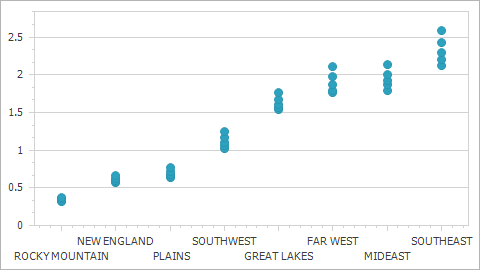Ensure that the measure unit is specified before you apply a summary function to series data. To illustrate this behavior, consider a chart control bound to the following data table.

SeriesPoint Argument Value
 1 5
 1 10
 2 20
 2 40
 3 20
 3 65
 5 15
 6 25
 7 50
 8 25
 9 65
 10 25
 10 15
 20 120
 20 120

The image below shows how the SUM summary function depends on the measure unit.

MeasureUnit = Ones, SummaryFunction = SUM MeasureUnit = Tens, SummaryFunction = SUM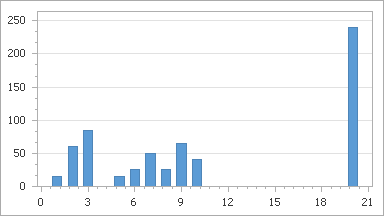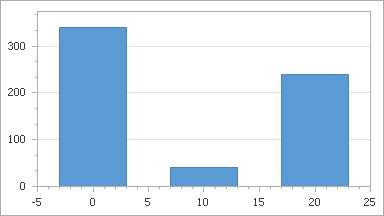In the charts above, the NumericScaleOptions.MeasureUnit (DateTimeScaleOptions.MeasureUnit or TimeSpanScaleOptions.MeasureUnit) property is used to specify measure units manually. To specify these units manually, note that the ScaleOptionsBase.ScaleMode property must be set to Manual.

The chart control can also calculate the measure unit and apply it to the X-axis automatically, based on the underlying data set. To calculate the measure unit automatically, set the ScaleOptionsBase.ScaleMode property to Automatic - to activate automatic scale mode. For more information, refer to the following help topic: Data Aggregation.

Note

If you specify the summary function, the aggregate function (ScaleGridOptionsBase.AggregateFunction) cannot be applied to data.

In addition to the built-in summary functions, you can also create your own custom function to calculate summaries. For more details, see the following help topic: Custom Summary Functions.

## Example

Consider the chart from Lesson 4 (see the “Create Data Objects and Bind a ChartControl” section), bound to the GSP database.

Add a Point series to the chart. Then, set the SeriesBase.ArgumentDataMember property to the Region data field, and SeriesBase.ValueDataMembers property to GSP.

This initial chart is shown below.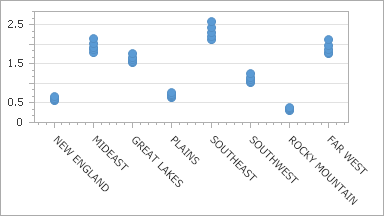To calculate the AVERAGE function for the GSP data field, do the following.

• Select the required series and in the Properties window, locate its SummaryFunction property.
• Click the property’s ellipsis button to invoke the Summary Function dialog. In the invoked dialog, choose the required AVERAGE summary function and the data field to be averaged.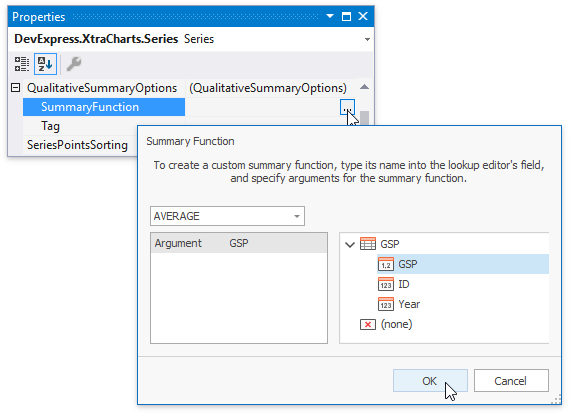• To apply the changes and quit the dialog, click OK.

The result is shown in the image below.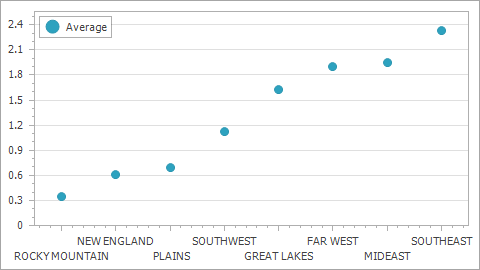The following code snippet illustrates how to do the same at runtime.

``````series1.QualitativeSummaryOptions.SummaryFunction = "AVERAGE([GSP])";
``````

## Custom Summary Functions

To create a custom summary function, register it by calling the WebChartControl.RegisterSummaryFunction method with the specified parameters (name, displayName, resultScaleType, resultDimensions, argumentDescriptions, function).

The code below illustrates how to do this in WinForms.

``````using System;
using System.Windows.Forms;
using DevExpress.XtraCharts;
// ...

namespace WindowsApplication1 {
public partial class Form1 : Form {

// Declare the Product summary function.
private static SeriesPoint[] CalcProductValue(Series series, object argument,
string[] functionArguments, DataSourceValues[] values) {

// Create an array of the resulting series points.
SeriesPoint[] points = new SeriesPoint[values.Length];

// Calculate the resulting series points as Price * Count.
for (int i = 0; i < values.Length; i++)
points[i] = new SeriesPoint(argument,
Convert.ToDouble(values[i][functionArguments]) *
Convert.ToDouble(values[i][functionArguments]));

// Return the result.
return points;
}

public Form1() {
InitializeComponent();

// Create argument descriptions for the summary function.
SummaryFunctionArgumentDescription argument1Description =
new SummaryFunctionArgumentDescription("Price", ScaleType.Numerical);
SummaryFunctionArgumentDescription argument2Description =
new SummaryFunctionArgumentDescription("Count", ScaleType.Numerical);

// Register the summary function in a chart.
chartControl1.RegisterSummaryFunction("PRODUCT", "PRODUCT", 1,
new SummaryFunctionArgumentDescription[] {
argument1Description, argument2Description },
CalcProductValue);

// Provide a datasource for the chart.
chartControl1.DataSource = nwindDataSet.Products;
}

private void Form1_Load(object sender, EventArgs e) {
// Load data into the 'nwindDataSet.Products' table.